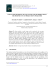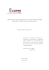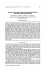# Lesson 21: Ptolemy`s Theorem

## Transcription

Lesson 21: Ptolemy`s Theorem
```Lesson 21
M5
GEOMETRY
Lesson 21: Ptolemy’s Theorem
Student Outcomes
Students determine the area of a cyclic quadrilateral as a function of its side lengths and the acute angle
formed by its diagonals.
Students prove Ptolemy’s theorem, which states that for a cyclic quadrilateral
. They explore applications of the result.
,
=
+
Lesson Notes
In this lesson, students work to understand Ptolemy’s theorem, which says that for a cyclic quadrilateral ,
=
+
. As such, this lesson focuses on the properties of quadrilaterals inscribed in circles.
Ptolemy’s single result and the proof for it codify many geometric facts; for instance, the Pythagorean theorem
(G-GPE.A.1, G-GPE.B.4), area formulas, and trigonometry results. Therefore, it serves as a capstone experience to our
year-long study of geometry. Students will use the area formulas they established in the previous lesson to prove the
theorem. A set square, patty paper, compass, and straight edge are needed to complete the Exploratory Challenge.
Classwork
Opening (2 minutes)
The Pythagorean theorem, credited to the Greek mathematician Pythagoras of Samos (ca. 570–ca. 495 BCE), describes a
universal relationship among the sides of a right triangle. Every right triangle (in fact every triangle) can be
circumscribed by a circle. Six centuries later, Greek mathematician Claudius Ptolemy (ca. 90–ca. 168 CE) discovered a
relationship between the side lengths and the diagonals of any quadrilateral inscribed in a circle. As we shall see,
Ptolemy’s result can be seen as an extension of the Pythagorean theorem.
Opening Exercise (5 minutes)
Students are given the statement of Ptolemy’s theorem and are asked to test the theorem by measuring lengths on
specific cyclic quadrilaterals they are asked to draw. Students conduct this work in pairs and then gather to discuss their
ideas afterwards in class as a whole. Remind students that careful labeling in their diagram is important.
Opening Exercise
Ptolemy’s theorem says that for a cyclic quadrilateral
=
+
.
MP.7
,
With ruler and a compass, draw an example of a cyclic quadrilateral. Label its
vertices , , , and .
Draw the two diagonals
and
Lesson 21:
.
Ptolemy’s Theorem
261
Lesson 21
M5
GEOMETRY
=
With a ruler, test whether or not the claim that
+
seems to hold true.
Repeat for a second example of a cyclic quadrilateral.
MP.7
Challenge: Draw a cyclic quadrilateral with one side of length zero. What shape is this cyclic quadrilateral? Does
Ptolemy’s claim hold true for it?
Students will see that the relationship
=
+
seems to hold, within measuring error. For a
quadrilateral with one side of length zero, the figure is a triangle inscribed in a circle. If the length
= , then the
points and coincide, and Ptolemy’s theorem states
=
+
, which is true.
Exploratory Challenge (30 minutes): A Journey to Ptolemy’s Theorem
This Exploratory Challenge will lead students to a proof of Ptolemy’s theorem. Students should work in pairs. The
teacher will guide as necessary.
Exploratory Challenge: A Journey to Ptolemy’s Theorem
with diagonals
and
intersecting to form an acute angle with degree measure .
= ,
= ,
= , and
= .
a.
From the last lesson, what is the area of quadrilateral
in terms of the lengths of its diagonals and the angle
? Remember this formula for later.
(
b.
)=
Explain why one of the angles,
measure less than or equal to
or
, has a
°.
Opposite angles of a cyclic quadrilateral are
supplementary. These two angles cannot both have
measures greater than ° (both angles could be equal to
c.
°).
Let’s assume that
in our diagram is the angle with a measure less than or
equal to °. Call its measure degrees. What is the area of triangle
in terms
of , , and ? What is the area of triangle
in terms of , , and ? What is the
in terms of , , , , and ?
If represents the degree measure of an acute angle,
then
would be the degree measure of angle
since opposite angles of a cyclic quadrilateral
are supplementary. The area of triangle
could
( ), and the area
then be calculated using
of triangle
could be calculated by
(
) , or
areas of triangles
and
following:
(
Lesson 21:
Ptolemy’s Theorem
)=
( ). So,
is the sum of the
, which provides the
+
.
Scaffolding:
For part (c) of the
Exploratory Challenge,
review Exercises 4 and 5
from Lesson 20 with a
small, targeted group,
which show that the area
formula for a triangle,
)=
Area(
sin( ),
can be used where
represents an acute angle.
to work through and
struggle with the
exploration on their own.
262
Lesson 21
M5
GEOMETRY
d.
We now have two different expressions representing the area of the same cyclic quadrilateral
seem to you that we are close to a proof of Ptolemy’s claim?
. Does it
Equating the two expressions gives us a relationship that does, admittedly, use the four side lengths of the
quadrilateral and the two diagonal lengths, but we also have terms that involve
( ) and
( ). These
terms are not part of Ptolemy’s equation.
In order to reach Ptolemy’s conclusion, in Exploratory Challenge, parts (e)–(j), students will use rigid motions to convert
to a new cyclic quadrilateral of the same area with the same side lengths (but in an
alternative order) and with its matching angle congruent to angle in the original diagram. Equating the areas of
these two cyclic quadrilaterals will yield the desired result. Again, have students complete this work in homogeneous
pairs or small groups. Offer to help students as needed.
e.
Trace the circle and points , , , and onto a sheet of patty paper. Reflect
triangle
about the perpendicular bisector of diagonal
. Let , , and
the images of the points , , and , respectively.
i.
What does the reflection do with points
be
and ?
Because the reflection was done about the perpendicular bisector of the diagonal
the diagonal are images of each other; i.e., = and = .
ii.
Is it correct to draw
Scaffolding:
The argument provided in part
(e), (ii) follows the previous
lesson. An alternative
argument is that the
perpendicular bisector of a
chord of a circle passes through
the center of the circle.
Reflecting a circle or points on
perpendicular bisector of the
chord is, therefore, a symmetry
of the circle; thus, must go to
a point on the same circle.
, the endpoints of
as on the circle? Explain why or why not.
Reflections preserve angle measure. Thus,
. Also,
is supplementary to
;
is too. This means that
is a quadrilateral with one pair (and, hence, both pairs) of
thus,
opposite angles supplementary. Therefore, it is cyclic. This means that lies on the circle that passes
through , , and . And this is the original circle.
iii.
has the same area as quadrilateral
.
Triangle
is congruent to triangle
by a congruence transformation, so these triangles have
the same area. It now follows that the two quadrilaterals have the same area.
Lesson 21:
Ptolemy’s Theorem
263
Lesson 21
M5
GEOMETRY
f.
The diagram shows angles having degree measures ,
, , , and .
Find and label any other angles having degree measures ,
See diagram. Justifications include the inscribed angle theorem and vertical angles.
g.
=
Explain why
+ in your diagram from part (f).
The angle with degree measure
It follows that = + .
h.
is an exterior angle to a triangle with two remote interior angles
Identify angles of measures , , , , and
and .
from part (e).
See diagram below.
i.
Write a formula for the area of triangle
in terms of , , and .
(
)=
( )
(
)=
( )
in terms of , , and
. Write a formula for the area of triangle
and
Lesson 21:
Ptolemy’s Theorem
264
Lesson 21
M5
GEOMETRY
j.
Based on the results of part (i), write a formula for the area of cyclic quadrilateral
, and .
(
k.
)=
(
)=
( )+
in terms of , , ,
( )
Going back to part (a), now establish Ptolemy’s theorem.
( )=
( ) (
=
)=
( )+
( )
( ) (
+
)
The two formulas represent the same area.
Distributive property
+
Multiplicative property of equality
or
=(
)+(
)
Substitution
Closing (3 minutes)
Gather the class together and ask the following questions:
What was most challenging in your work today?
Answers will vary. Students might say that it was challenging to do the algebra involved or to keep
track of congruent angles, for example.
Are you convinced that this theorem holds for all cyclic quadrilaterals?
Answers will vary, but students should say “yes.”
Will Ptolemy’s theorem hold for all quadrilaterals? Explain.
At present, we do not know. The proof seemed very specific to cyclic quadrilaterals, so we might
suspect it holds only for these types of quadrilaterals. (If there is time, students can draw an example of
non-cyclic quadrilateral and check that the result does not hold for it.)
Lesson Summary
THEOREM:
PTOLEMY’S THEOREM: For a cyclic quadrilateral
,
=
+
.
Relevant Vocabulary
CYCLIC QUADRILATERAL: A quadrilateral with all vertices lying on a circle is known as a cyclic quadrilateral.
Exit Ticket (5 minutes)
Lesson 21:
Ptolemy’s Theorem
265
Lesson 21
M5
GEOMETRY
Name
Date
Lesson 21: Ptolemy’s Theorem
Exit Ticket
What is the length of the chord
Lesson 21:
Ptolemy’s Theorem
266
Lesson 21
M5
GEOMETRY
Exit Ticket Sample Solutions
What is the length of the chord
Chord
, and
is a diameter of the circle because it makes a right angle with chord
+
=
.
=
=
By Ptolemy’s theorem:
+
=
, giving
.
Problem Set Sample Solutions
1.
An equilateral triangle is inscribed in a circle. If is a point on the circle, what does Ptolemy’s theorem have to say
about the distances from this point to the three vertices of the triangle?
It says that the sum of the two shorter distances is equal to the longer
distance.
2.
Kite
is inscribed in a circle. The kite has an area of
. ., and the ratio of the lengths of the noncongruent adjacent sides is
. What is the perimeter of the kite?
=
=
Since
=
+
=
Let
=
and
=
=
, then
+
=
=
.
be the length of
, then
=
=
=
= .
The length
=
= , and the length
Lesson 21:
Ptolemy’s Theorem
=
=
. Therefore, the perimeter of kite
is
.
267
Lesson 21
M5
GEOMETRY
3.
Draw a right triangle with leg lengths and and hypotenuse length . Draw a rotated copy of the triangle such
+
We get
4.
, the Pythagorean theorem.
Draw a regular pentagon of side length in a circle. Let be the length of its diagonals. What does Ptolemy’s
theorem say about the quadrilateral formed by four of the vertices of the pentagon?
=
5.
=
+ , so
+
=
. (This is the famous golden ratio!)
The area of the inscribed quadrilateral is
Since
is a rectangle, then
diameters of the circle.
=
The length of
=
(
=
, and the length of
(
=
, and
. Determine the circumference of the circle.
, and the diagonals are
=
, so
).
)=
=
=
=
=
+
=
+
=
+
=
=
=
Since
=
, the radius of the circle is
Lesson 21:
Ptolemy’s Theorem
, and the circumference of the circle is
.
268
Lesson 21
M5
GEOMETRY
6.
Extension: Suppose and are two acute angles, and the circle has a diameter of unit. Find , , , and
terms of and . Apply Ptolemy’s theorem, and determine the exact value of
( °).
in
Use scaffolded questions below as needed.
a.
Explain why
( )
equals the diameter of the circle.
If the diameter is , this is a right triangle because it is inscribed
( ) = , or =
in a semicircle, so
. Since the diameter
( )
is , the diameter is equal to
b.
If the circle has a diameter of , what is ?
=
c.
( )
.
( )
Use Thales’ theorem to write the side lengths in the original diagram in terms of
and .
Since both are right triangles, the side lengths are
( ), =
( ), =
( ), and =
( ).
=
d.
If one diagonal of the cyclic quadrilateral is , what is the other?
( + )
e.
What does Ptolemy’s theorem give?
( + )=
f.
( )
( )+
( )
( )
(
Using the result from part (e), determine the exact value of
(
)=
(
Lesson 21:
+
)=
(
)
Ptolemy’s Theorem
(
)+
(
)
(
°).
)=
+
=
+
269
```

### Almagest, Book I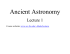### ASTRONOMY 161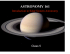### A family of newforms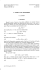### Ptolemy`s World Map and Eratosthenes`s Circumference of the Earth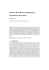### Ptolemy`s cosmology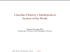### COORDINATE GEOMETRY Proofs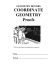### 5a: So, what was wrong with Ptolemy`s model to a contemporary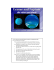### Finding Zeroes of Maps: Homotopy Methods That### nth - FLYPARSONS.org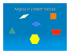### Lecture #4 - History of Astronomy - Ptolemy to Kepler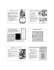### Chapter 3: Algebra: Real Numbers and the Pythagorean Theorem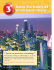### Discovering the Pythagorean Theorem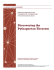### f - Departamento de Matematica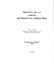### Proving the Pythagorean Theorem - the Mathematics Assessment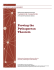### Analysis qualifying exam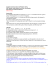### Alf van der Poorten and Continued Fractions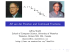### Descriptive Set Theory and Harmonic Analysis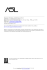### SIROCCO 2016 - Daniel Graf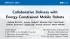### Chapter 3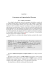### UBLUE FOR THE REGULAR TWO-STAGE LINEAR MODEL FROM